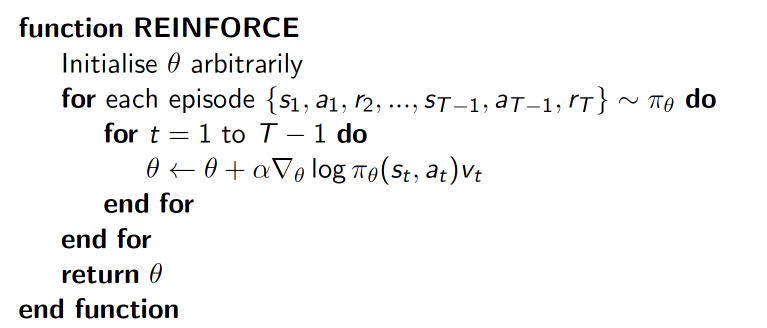## 要点 ¶

Policy gradient 是 RL 中另外一个大家族, 他不像 Value-based 方法 (Q learning, Sarsa), 但他也要接受环境信息 (observation), 不同的是他要输出不是 action 的 value, 而是具体的那一个 action, 这样 policy gradient 就跳过了 value 这个阶段. 而且个人认为 Policy gradient 最大的一个优势是: 输出的这个 action 可以是一个连续的值, 之前我们说到的 value-based 方法输出的都是不连续的值, 然后再选择值最大的 action. 而 policy gradient 可以在一个连续分布上选取 action.

## 算法 ¶delta(log(Policy(s,a))*V) 表示在 状态 s 对所选动作 a 的吃惊度, 如果 Policy(s,a) 概率越小, 反向的 log(Policy(s,a)) (即 -log(P)) 反而越大. 如果在 Policy(s,a) 很小的情况下, 拿到了一个 大的 R, 也就是 大的 V, 那 -delta(log(Policy(s, a))*V) 就更大, 表示更吃惊, (我选了一个不常选的动作, 却发现原来它能得到了一个好的 reward, 那我就得对我这次的参数进行一个大幅修改). 这就是吃惊度的物理意义啦.

## 算法代码形式 ¶

import gym
import matplotlib.pyplot as plt

RENDER = False  # 在屏幕上显示模拟窗口会拖慢运行速度, 我们等计算机学得差不多了再显示模拟
DISPLAY_REWARD_THRESHOLD = 400  # 当 回合总 reward 大于 400 时显示模拟窗口

env = gym.make('CartPole-v0')   # CartPole 这个模拟
env = env.unwrapped     # 取消限制
env.seed(1)     # 普通的 Policy gradient 方法, 使得回合的 variance 比较大, 所以我们选了一个好点的随机种子

print(env.action_space)     # 显示可用 action
print(env.observation_space)    # 显示可用 state 的 observation
print(env.observation_space.high)   # 显示 observation 最高值
print(env.observation_space.low)    # 显示 observation 最低值

# 定义
n_actions=env.action_space.n,
n_features=env.observation_space.shape,
learning_rate=0.02,
reward_decay=0.99,   # gamma
# output_graph=True,    # 输出 tensorboard 文件
)


for i_episode in range(3000):

observation = env.reset()

while True:
if RENDER: env.render()

action = RL.choose_action(observation)

observation_, reward, done, info = env.step(action)

RL.store_transition(observation, action, reward)    # 存储这一回合的 transition

if done:
ep_rs_sum = sum(RL.ep_rs)

if 'running_reward' not in globals():
running_reward = ep_rs_sum
else:
running_reward = running_reward * 0.99 + ep_rs_sum * 0.01
if running_reward > DISPLAY_REWARD_THRESHOLD: RENDER = True     # 判断是否显示模拟
print("episode:", i_episode, "  reward:", int(running_reward))

vt = RL.learn() # 学习, 输出 vt, 我们下节课讲这个 vt 的作用

if i_episode == 0:
plt.plot(vt)    # plot 这个回合的 vt
plt.xlabel('episode steps')
plt.ylabel('normalized state-action value')
plt.show()
break

observation = observation_